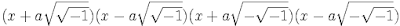## Monday, December 10, 2007

### Leibniz's Mistake

In 1702, Gottfried Leibniz wrote an important paper on the integration of rational fractions. It was well known that the time that ∫ dx/x = log x (see Lemma 1, here for proof) and Leibniz had previously discovered in 1675 that ∫ dx/(x2 + 1) = tan-1x (see Theorem, here).

Leibniz knew that if a polynomial could be decomposed into irreducible parts, then a rational fraction could be restated as the sum of partial fractions (see here for details).

In particular, Leibniz tried to figure out if all polynomials were decomposable into dx/x and dx/(x2 + 1). In answer, Leibniz believed that he had found a polynomial form that resisted any such attempt at decomposition.

Leibniz noted that:

x4 + a4 = (x2 + a2-1)(x2 + a2-1) =Leibniz then concludes that:

"Therefore, ∫ dx/(x4 + a4) cannot be reduced to the squaring of the circle [ dx/(x2 + 1)] or the hyperbola [ dx/x ] by our analysis ..., but founds a new kind of its own." [see Tignol, p 75]

In short, Leibniz did not understand roots of unity. He did not realize that:His mistake would later be corrected by Roger Cotes whose response to Leibniz will be the next part in our story of the roots of unity.

In 1719, N. Bernoulli put forward the following response to Leibniz:

x4 + a4 = (x2 + a2)2 - 2a2x2 =

= (x2 + a2 + √2ax)(x2 + a2 - √2ax)

In retrospect, Leibniz's mistake led to the clarification of the roots of unity.

References
• Jean-Pierre Tignol, Galois' Theory of Algebraic Equations, World Scientific, 2001